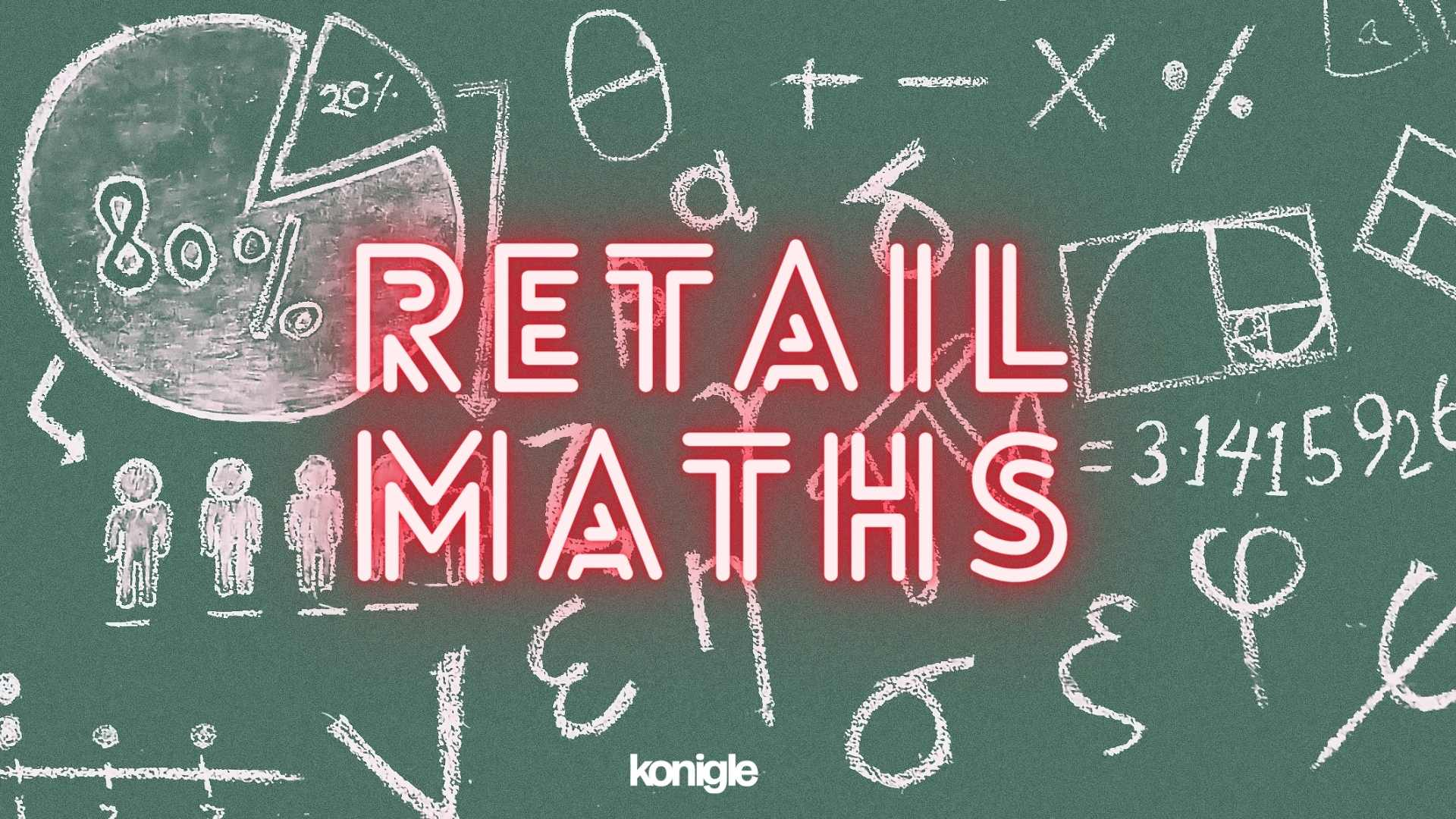### Retail Math: Fundamentals & FormulaExcelling at e-commerce requires making decisions based on gut instinct, experience, and logic. Retail math concepts such as ratios, percentage change, probability, and inequalities can help us make better decisions and achieve profit growth.

Excelling at e-commerce needs you to make many decisions. Most of our decisions are taken based on

1. Gut Instinct :  Usually the decision feels right and we take it.  But our gut instinct could be negatively influenced by our mood, state of mind and recent happenings around us. But positively by our logical thinking.
2. Experience :  A lot of our decisions are also influenced by experience, both good experiences as well as bad. And both these experiences may lead us to make incorrect decisions out of both irrational exuberance or fear.
3. Logic :  Coming to a decision logically is both a science and an art. As making decisions logically could also lead sometimes to get into an analysis paralysis situation leading to suboptimal decisions.

The reality is,  that taking a correct decision usually is a combination of the three above backed by being able to use mathematics 🧮  to measure or predict outcomes. Hence, we decided to take a short trip back into basic retail math to help revise fundamental mathematics concepts to help us take better decisions and excel at e-commerce.

### Ratio

Ratios help us understand the relationship between different quantities. They can be used to compare things, like the number of boys to girls in a class or the number of apples to oranges in a basket. In a retail context a Ratio can be used to compare quantity of product sold to the quantity of product bought for each SKU, this ratio is commonly used to define sell through.

### Percentage change

Understanding percentage change is not as easy as it seems but one of the most often used mathematics concepts in commerce . Hence, we previously wrote about it in Calculating 10% off a price: Easy or Tough ? .

Imagine there's a fashion store that is having a flash sale across their entire website for BFCM. The original price of a t-shirt is \$50, but during the flash sale, it is discounted to \$40. We can calculate the percentage change to see how much the price has decreased.

First, we find the difference between the original price and the discounted price: \$50 - \$40 = \$10. Then, we take that difference (\$10) and divide it by the original price (\$50): \$10 / \$50 = 0.2. Finally, we multiply the result by 100 to get the percentage: 0.2 * 100 = 20%.

So, the percentage change is 20%. This means that during the flash sale, the price of the t-shirt has decreased by 20%.

In the example below, a Shopify store is using the Konigle Bulk Price Editor to reduce prices of 9 t-shirt SKUs by 10\$ and then run a flash sale.

### Probability

Now, let's talk about probability. Probability is a way to understand how likely something is to happen. Let's consider an example where a retail store wants to forecast the probability of selling a particular product during a specific time period, let's say a week.

The store has historical data that shows that out of the total number of customers who visit the store during a week, 20% of them purchase the specific product. The store wants to estimate the probability of selling the product to a customer who walks into the store in the coming week.

In this case, the probability of selling the product can be determined by analyzing the historical data. If, on average, 20 out of every 100 customers purchase the product, the probability of a customer making a purchase can be estimated as 20%.

By understanding the probability, the retail store can make informed decisions about inventory management, marketing strategies, and resource allocation. For example, if they forecast a higher probability of selling the product, they might stock up on more inventory.

### Inequalities

Inequalities are a mathematical way to compare numbers or quantities and help us make decisions based on different criteria. In a online store, when we want to filter or select products based on their stock levels, we use inequalities.

For example, let's say we want to find all the products that have more than 10 items in stock. We can write this as "stock > 10." It means we are looking for products where the stock level is greater than 10.

Similarly, if we want to find products that have less than or equal to 5 items in stock, we can write it as "stock ≤ 5." It means we are looking for products where the stock level is either equal to or less than 5.

Moreover, we may want to run a promotion for products that have more than 5 and less than 50 items in stock, this inequality is expressed as 5 < x < 50. In fact, a Shopify store can quickly filter products by inventory levels and run a promotion using the Konigle Bulk Price Editor.

I hope this brief revision of retail math concepts can help excel at e-commerce and have profit growth.